# Lossless Decomposition in DBMS

DBMS | Lossless Decomposition: Here, we are going to learn about the Lossless Decomposition, rule for Lossless Decomposition, its properties.
Submitted by Anushree Goswami, on June 29, 2019

Lossless decomposition occurs when the natural join of the decomposition of relation R gives exactly the same result as R. Let us consider the decomposition of relation R as A, B, and C.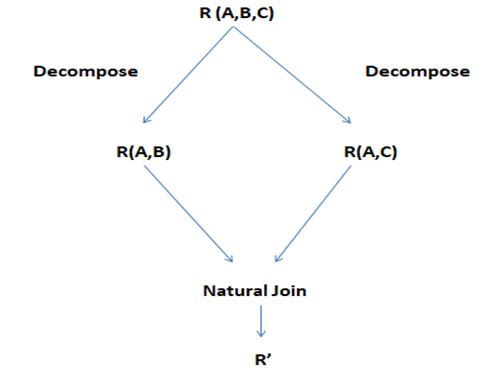Here, if R'= R, it is Lossless Decomposition.

## Rule for Lossless Decomposition

1. There must be functional dependency relationship in between the attributes of the relation, i.e., if A, B, and C are the attributes of the relation R and we decompose the relation into R (A, B) and R (A, C), then A → B(B attribute depends on A attribute) and A → C(C attribute depend on A attribute). This means that the relationship is having lossless decomposition.
2. Only if the above condition holds, then after the natural join of decomposed relations of R, R (A, B) and R(A, C), the new relation obtained, R' will be equal to R. This shows that the relationship is having lossless decomposition.

### Properties of Lossless Decomposition

Let us consider relation as R and set of Functional Dependency on R as F.
A, B: Attributes of R.
The, decomposition is lossless if,

1. A ∩ B → A, which means all the attributes that are common on both A & B functionally determines all the attributes in A.
2. A ∩ B → B, which means all the attributes that are common on both A & B functionally determine all the attributes in B.
3. A ∩ B forms on the super key of either A or B, then also the decomposition of R is lossless.

For e.g. – Table R: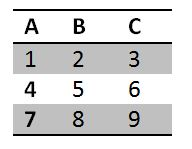Decomposition of Relation into R (A, B) and R (A, C), we get -

R (A, B) -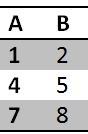R (A, C) -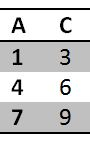Now, Doing Natural Join of R (A, B) and R (A, C), we get -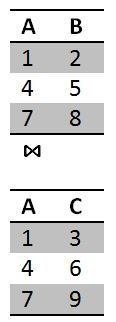= R'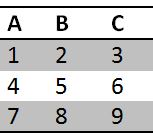Here, R' = R, this shows that relation R is Lossless Decomposition.

What's New

Top Interview Coding Problems/Challenges!

Languages: » C » C++ » C++ STL » Java » Data Structure » C#.Net » Android » Kotlin » SQL
Web Technologies: » PHP » Python » JavaScript » CSS » Ajax » Node.js » Web programming/HTML
Solved programs: » C » C++ » DS » Java » C#
Aptitude que. & ans.: » C » C++ » Java » DBMS
Interview que. & ans.: » C » Embedded C » Java » SEO » HR
CS Subjects: » CS Basics » O.S. » Networks » DBMS » Embedded Systems » Cloud Computing
» Machine learning » CS Organizations » Linux » DOS
More: » Articles » Puzzles » News/Updates